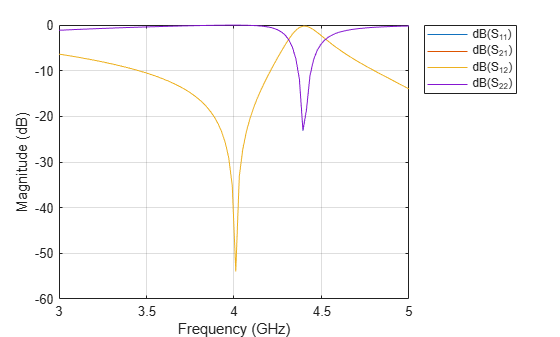Create single- and double-radial stub shunt on X-Y plane

Since R2021b

## Description

Use the `stubRadialShunt` object to create a single- or double-radial stub shunt on the X-Y plane.Radial stubs provide broadly resonant RF short circuits by introducing the short at a concentrated point. When cascaded with high-impedance quarter-wavelength transmission lines, radial stubs provide an effectively decoupled network for microwave amplifiers and other active components.

## Creation

### Syntax

``stub = stubRadialShunt``
``stub = stubRadialShunt(Name=Value)``

### Description

example

````stub = stubRadialShunt` creates a single-radial stub shunt in the X-Y plane. The stub dimensions are for the frequency range of 3-5 GHz with a resonant frequency of 4.2 GHz on the X-Y plane.```

example

````stub = stubRadialShunt(Name=Value)` sets Properties using one or more name-value arguments. For example, `stubRadialStub(OuterRadius=0.0070)` creates a radial stub shunt with an outer radius of `0.0070` meters. Properties not specified retain their default values.```

## Properties

expand all

Type of radial stub, specified as `"Single"` or `"Double"`.

Example: `stub = stubRadialShunt(StubType="Double")`

Data Types: `string` | `char`

Outer radius of the radial stub in meters, specified as a positive scalar or a two-element vector of positive elements. Specify a two-element vector for a double-radial stub.

Example: `stub = stubRadialShunt(OuterRadius=0.0070)`

Data Types: `double`

Inner radius of the radial stub in meters, specified as a positive scalar or a two-element vector of positive elements. Specify a two-element vector for a double-radial stub.

Example: `stub = stubRadialShunt(InnerRadius=0.0023)`

Data Types: `double`

Angle of the stub in degrees, specified as a positive scalar or a two-element vector of positive elements. Specify a two-element vector for a double-radial stub. The stub angles must be greater than or equal to `5` degrees and less than or equal to `175` degrees.

Example: `stub = stubRadialShunt(Angle=60)`

Data Types: `double`

Width of the microstrip line in meters, specified as a positive scalar.

Example: `stub = stubRadialShunt(PortLineWidth=0.0035)`

Data Types: `double`

Length of the microstrip line in meters, specified as a positive scalar.

Example: `stub = stubRadialShunt(PortLineLength=0.0237)`

Data Types: `double`

Height of the radial stub from the ground plane, specified as a positive scalar.

Example: `stub = stubRadialShunt(Height=0.0015)`

Data Types: `double`

Length of the ground plane in meters, specified as a positive scalar.

Example: `stub = stubRadialShunt(GroundPlaneLength=0.046)`

Example: double

Width of the ground plane in meters, specified as a positive scalar.

Example: `stub = stubRadialShunt(GroundPlaneWidth=0.046)`

Example: double

Type of dielectric material used as a substrate, specified as a `dielectric` object. The dielectric material in a `stubRadialShunt` object with default properties is Teflon. The thickness of the default dielectric material Teflon is `0.8` mm or the same as the `Height` property.

Example: `d = dielectric("FR4");` `stub = stubRadialShunt(Substrate=d)`

Data Types: `string` | `char`

Type of metal used in the conducting layers, specified as a `metal` object. The type of metal in a `stubRadialShunt` object with default properties is PEC.

Example: `m = metal("PEC");` `stub = stubRadialShunt(Conductor=m)`

Data Types: `string` | `char`

Flag to add a metal shielding to the PCB component, specified as a logical 0 or logical 1.

Example: `IsShielded = true` or `1` add a metal shield.

Note

To enable FEM solver required for the metal shield property, download the `Integro-Differential Modeling Framework for MATLAB`. To download this add-on:

1. In the Home tab Environment section, click on . This opens the add-on explorer. You need an active internet connection to download the add-on.

2. Search for ```Integro-Differential Modeling Framework for MATLAB``` and click .

`matlab.addons.installedAddons`
in your MATLAB® session command line.

Data Types: `logical`

Metal shield for the PCB component, specified as a `shape.Box` object. The length and width of the box must be equal to the length and width of the ground plane. The center of the box is at `[0 0 Height]`. You can modify the property after creating the object.

#### Dependencies

To enable the `Shielding` property, set the `IsShielded` property to `true` or `1`.

Type of RF connector assembled at the feed locations of the PCB component, specified as a `RFConnector` object.

Example: Create connector from `RFConnector` object like this: `coaxial = RFConnector` adds a coaxial connector.

#### Dependencies

To enable the `Connector` property, set the `IsShielded` property to `true` or `1`.

## Object Functions

 `charge` Calculate and plot charge distribution `current` Calculate and plot current distribution `feedCurrent` Calculate current at feed port `getZ0` Calculate characteristic impedance of transmission line `layout` Plot all metal layers and board shape `mesh` Change and view mesh properties of metal or dielectric in PCB component `shapes` Extract all metal layer shapes of PCB component `show` Display PCB component structure or PCB shape `sparameters` Calculate S-parameters for RF PCB objects `RFConnector` Create RF connector

## Examples

collapse all

Create a default radial stub shunt.

`stub = stubRadialShunt`
```stub = stubRadialShunt with properties: StubType: 'Single' OuterRadius: 0.0085 InnerRadius: 0.0012 Angle: 90 PortLineLength: 0.0137 PortLineWidth: 0.0025 Height: 8.0000e-04 GroundPlaneLength: 0.0200 GroundPlaneWidth: 0.0200 Substrate: [1x1 dielectric] Conductor: [1x1 metal] IsShielded: 0 ```

`show(stub)`Create shunt radial stub of type double.

```stub = stubRadialShunt(StubType='double'); stub.OuterRadius = [0.0085 0.0065]; stub.InnerRadius = [0.0012 0.0008]; stub.Angle = [90 60];```

`show(stub)`Plot s-parameters.

```spar = sparameters(stub,linspace(3e9,5e9,50)); rfplot(spar)```